# Linear Programming Introduction What is Linear Programming A

• Slides: 36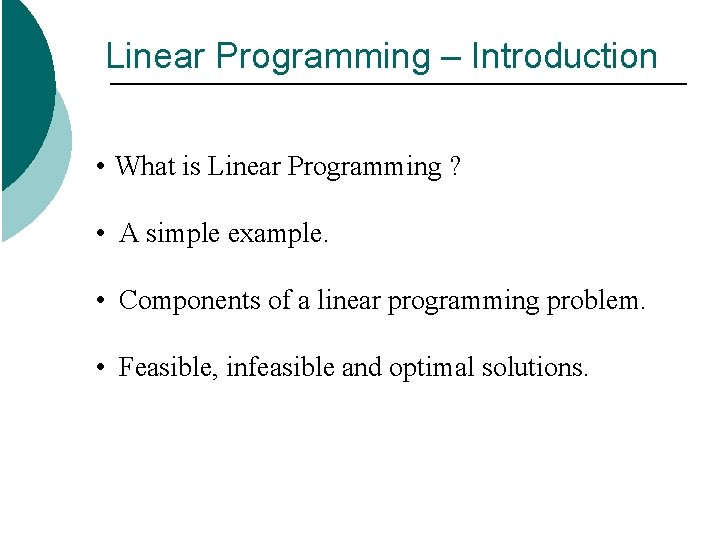Linear Programming – Introduction • What is Linear Programming ? • A simple example. • Components of a linear programming problem. • Feasible, infeasible and optimal solutions.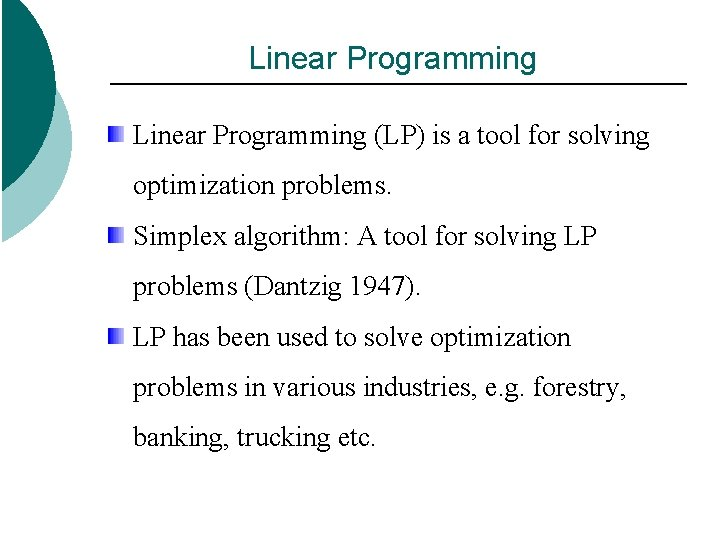Linear Programming (LP) is a tool for solving optimization problems. Simplex algorithm: A tool for solving LP problems (Dantzig 1947). LP has been used to solve optimization problems in various industries, e. g. forestry, banking, trucking etc.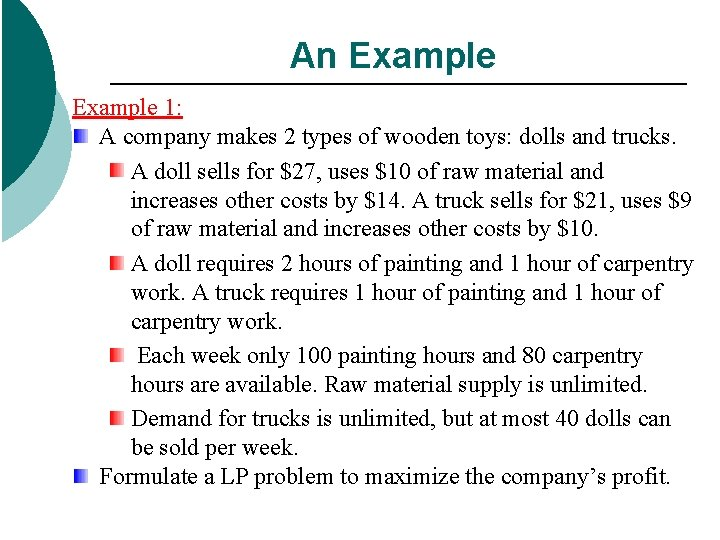An Example 1: A company makes 2 types of wooden toys: dolls and trucks. A doll sells for \$27, uses \$10 of raw material and increases other costs by \$14. A truck sells for \$21, uses \$9 of raw material and increases other costs by \$10. A doll requires 2 hours of painting and 1 hour of carpentry work. A truck requires 1 hour of painting and 1 hour of carpentry work. Each week only 100 painting hours and 80 carpentry hours are available. Raw material supply is unlimited. Demand for trucks is unlimited, but at most 40 dolls can be sold per week. Formulate a LP problem to maximize the company’s profit.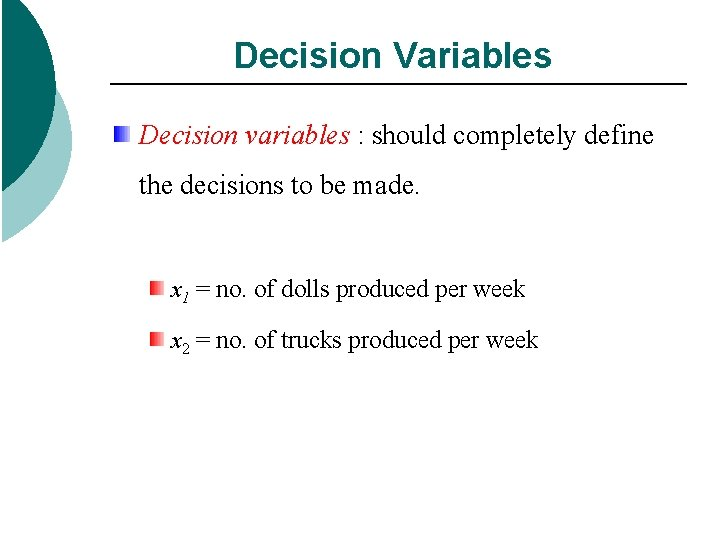Decision Variables Decision variables : should completely define the decisions to be made. x 1 = no. of dolls produced per week x 2 = no. of trucks produced per weekObjective Function In any LP problem the decision maker wants to maximize (usually revenue or profit) or minimize (usually costs) some function of the decision variables. The function to be maximized or minimized is called the objective function. What is the objective in Example 1? Maximize profit = revenues – costs Costs Fixed costs: does not depend on decision variables (e. g. rent) Variable costs: raw material costs + other variable costs. Revenues weekly revenues from dolls + weekly revenues from trucks.Objective Function Revenues = weekly revenues from dolls + weekly revenues from trucks. = (selling price per doll * no. of dolls per week) + (selling price per truck * no. of trucks per week) = 27*x 1 + 21*x 2.Objective Function Weekly raw material costs = 10 x 1 + 9 x 2 Other weekly variable costs = = 14 x 1 + 10 x 2 So, profits = (27 x 1 + 21 x 2) – (10 x 1 +9 x 2) – (14 x 1 + 10 x 2) = 3 x 1 +2 x 2 Therefore, objective function = Maximize z = 3*x 1 +2*x 2 The coefficient of a decision variable in the objective function is called an objective function coefficient. The variable z is used to represent the objective function value of any LP.Constraints : restrictions that limit the values of the decision variables. Constraints for Example 1: c 1) No more than 100 hours of painting time can be used per week. c 2) No more than 80 hours of carpentry time can be used per week. c 3) Due to limited demand, at most 40 dolls should be produced per week.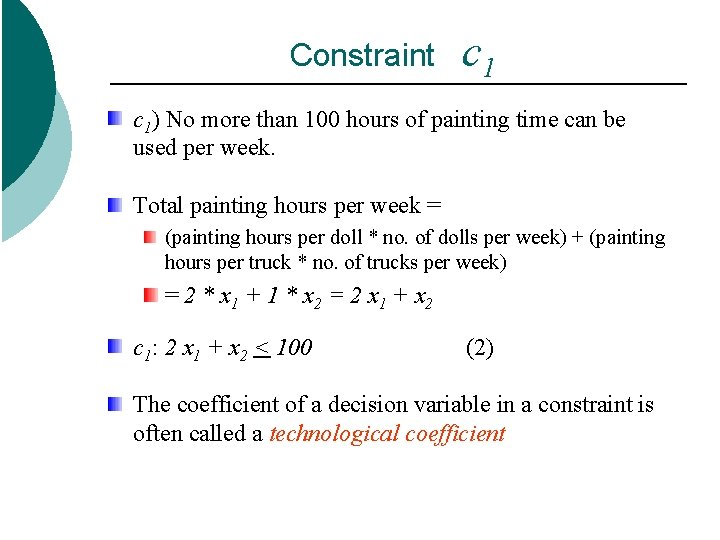Constraint c 1) No more than 100 hours of painting time can be used per week. Total painting hours per week = (painting hours per doll * no. of dolls per week) + (painting hours per truck * no. of trucks per week) = 2 * x 1 + 1 * x 2 = 2 x 1 + x 2 c 1: 2 x 1 + x 2 < 100 (2) The coefficient of a decision variable in a constraint is often called a technological coefficientConstraint c 2) No more than 80 hours of carpentry time can be used per week. Total carpentry hours per week = (carpentry hours per doll * no. of dolls per week) + (carpentry hours per truck * no. of trucks per week) = 1 * x 1 + 1 * x 2 = x 1 + x 2 c 2: x 1 + x 2 < 80 (3)Constraint c 3) Due to limited demand, at most 40 dolls should be produced per week. No. of dolls per week = x 1 Maximum no. of dolls produced per week = 40 c 3: x 1 < 40 (4)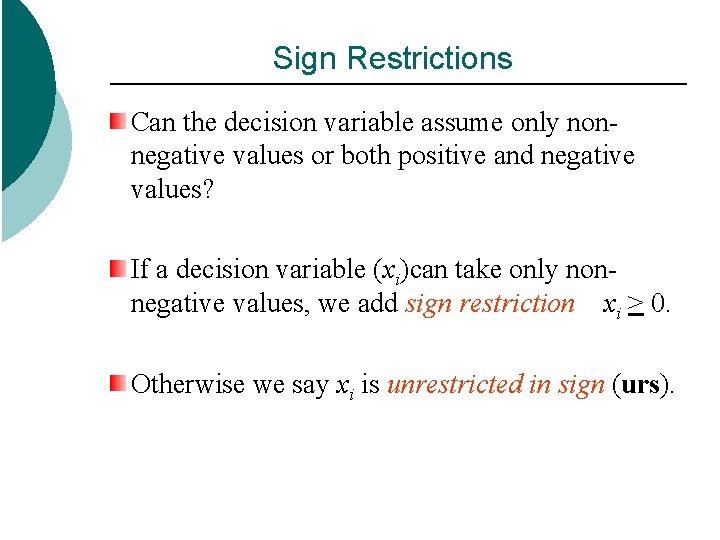Sign Restrictions Can the decision variable assume only nonnegative values or both positive and negative values? If a decision variable (xi)can take only nonnegative values, we add sign restriction xi > 0. Otherwise we say xi is unrestricted in sign (urs).LP Formulation for Example 1 Maximize z = 3*x 1 +2*x 2 (1) Objective function subject to (s. t. ) 2*x 1 + x 2 < 100 (2) painting constraint x 1 + x 2 < 80 (3) carpentry constraint x 1 x 2 (4) (5) (6) demand constraint < 40 > 0 sign restrictionSome Definitions Definition 1: A function f(x 1, x 2, …, xn) of x 1, x 2, …, xn is a linear function if and only if for some set of constants c 1, c 2, …, cn, f(x 1, x 2, …, xn) = c 1 x 1+c 2 x 2+…+cnxn For example, f(x 1, x 2) = 2 x 1 + x 2 is a linear function of x 1 and x 2, but f(x 1, x 2) = x 12 x 2 is not a linear function of x 1 and x 2 Definition 2: For any linear function f and any number b, the inequalities f(x 1, x 2, …, xn) ≤ b and f(x 1, x 2, …, xn) ≥ b are linear inequalities. Thus, 2 x 1 + 3 x 2 ≤ 3 and 2 x 1 + x 2 ≥ 3 are linear inequalities, but x 12 x 2≥ 3 is not a linear inequality.Some Definitions (cont’d) Definition 3: A linear programming problem (LP) is an optimization problem for which we do the following: 1. We attempt to maximize ( or minimize) a linear function of the decision variables. The function that is to be maximized or minimized is called the objective function. 2. The values of the decision variables must satisfy a set of constraints. Each constraint must be a linear equation or linear inequality. 3. A sign restriction is associated with each variable. For any variable xi, the sign restriction specifies either that xi must be nonnegative ( xi ≥ 0) or that xi may be unrestricted in sign (urs)Feasible Region The feasible region for an LP is the set of points satisfying all its constraints and sign restrictions. Is the point (x 1 = 40, x 2 = 20 ) in the feasible region for Example 1: Put these values (of x 1 and x 2) in each constraint (2)-(6) and check if the constraint is satisfied for each case. Ans: (x 1 = 40, x 2 = 20 ) is in the feasible region. Is the point (x 1 = 15, x 2 = 70 ) in the feasible region of Example 1: No, it violates (3) Is the point (x 1 = 40, x 2 = -20 ) in the feasible region of Example 1: No, it violates (6), i. e. sign restriction on x 2. Any point that is not in an LP’s feasible region is called an infeasible point.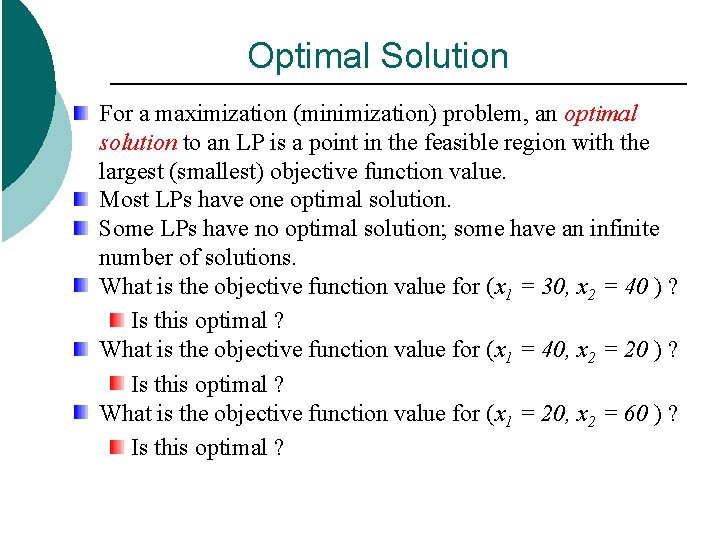Optimal Solution For a maximization (minimization) problem, an optimal solution to an LP is a point in the feasible region with the largest (smallest) objective function value. Most LPs have one optimal solution. Some LPs have no optimal solution; some have an infinite number of solutions. What is the objective function value for (x 1 = 30, x 2 = 40 ) ? Is this optimal ? What is the objective function value for (x 1 = 40, x 2 = 20 ) ? Is this optimal ? What is the objective function value for (x 1 = 20, x 2 = 60 ) ? Is this optimal ?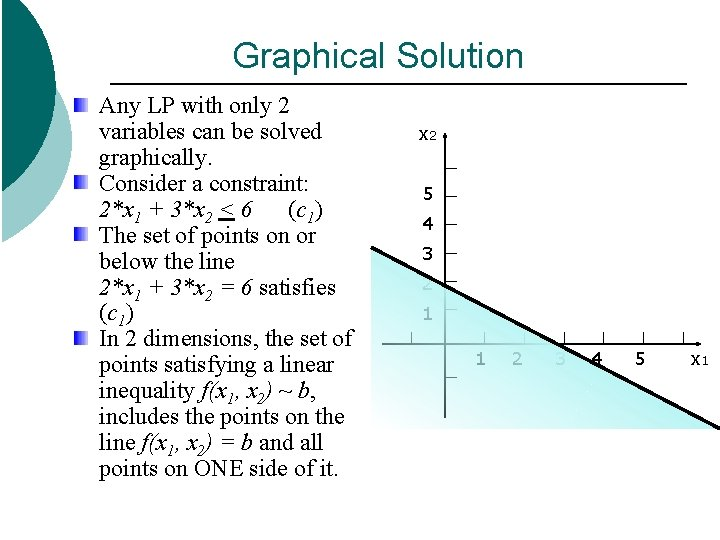Graphical Solution Any LP with only 2 variables can be solved graphically. Consider a constraint: 2*x 1 + 3*x 2 < 6 (c 1) The set of points on or below the line 2*x 1 + 3*x 2 = 6 satisfies (c 1) In 2 dimensions, the set of points satisfying a linear inequality f(x 1, x 2) ~ b, includes the points on the line f(x 1, x 2) = b and all points on ONE side of it. x 2 5 4 3 2 1 1 2 3 4 5 x 1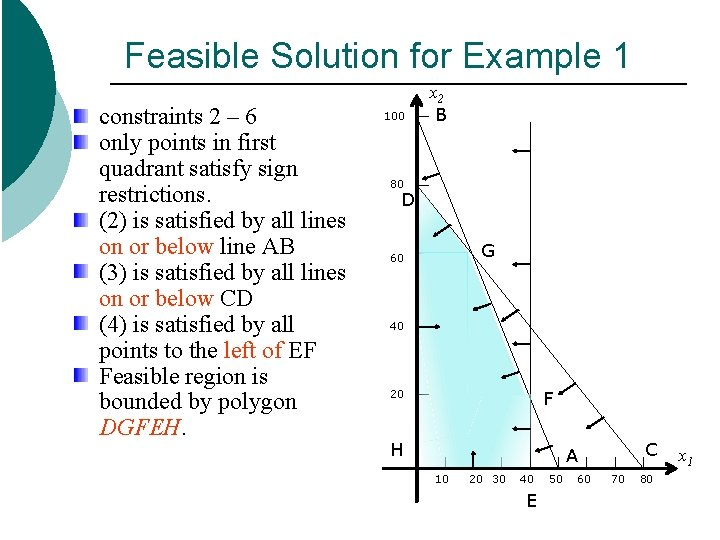Feasible Solution for Example 1 constraints 2 – 6 only points in first quadrant satisfy sign restrictions. (2) is satisfied by all lines on or below line AB (3) is satisfied by all lines on or below CD (4) is satisfied by all points to the left of EF Feasible region is bounded by polygon DGFEH. 100 x 2 B 80 D G 60 40 F 20 H C A 10 20 30 40 E 50 60 70 80 x 1Optimal Solution for Example 1 Optimal solution is the point in the feasible region with the largest value of z=3 x 1+2 x 2 Draw a single isoprofit (isocost for min problem) line, z =60 Generate other isoprofit lines by moving parallel to the drawn isoprofit line, such as z=100 Point G is the last isoprofit line to intersect the feasible region. And the optimal value is 180 100 x 2 B 80 D G 60 40 F 20 E 10 20 30 Z = 60, C A 40 50 100, 60 70 180 80 x 1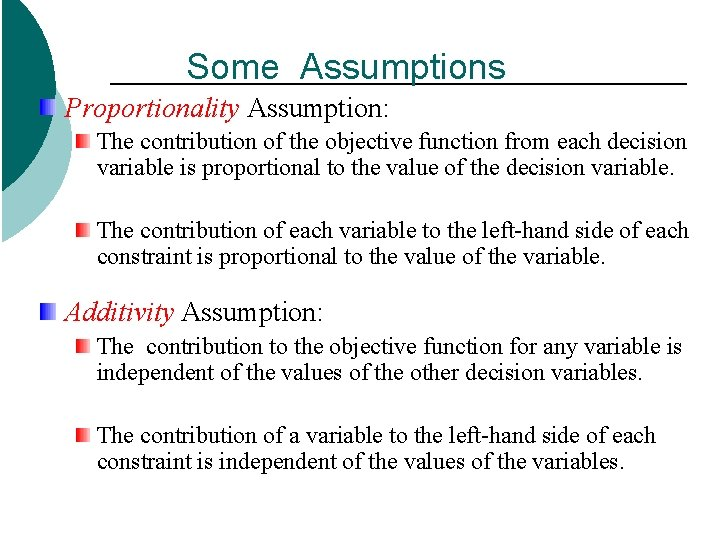Some Assumptions Proportionality Assumption: The contribution of the objective function from each decision variable is proportional to the value of the decision variable. The contribution of each variable to the left-hand side of each constraint is proportional to the value of the variable. Additivity Assumption: The contribution to the objective function for any variable is independent of the values of the other decision variables. The contribution of a variable to the left-hand side of each constraint is independent of the values of the variables.Some Assumptions (cont’d) Divisibility Assumption: each decision variable allowed to assume fractional values E. g. , in Example 1, it implies that it is acceptable to produce 1. 5 dolls, or 1. 63 trucks. If divisibility is not valid, rounding off each variable in the optimal LP solution to an integer may yield a reasonable solution. Certainty Assumption: each parameter ( objective function coefficient, right-hand side, and technological coefficient ) is known with certainty. E. g if we were unsure of the exact amount of carpentry hours required to build a truck, the Certainty Assumption would be violated.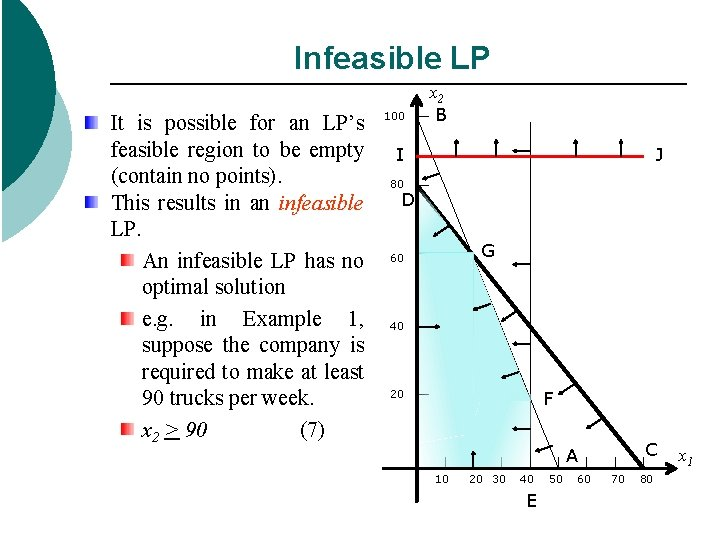Infeasible LP It is possible for an LP’s feasible region to be empty (contain no points). This results in an infeasible LP. An infeasible LP has no optimal solution e. g. in Example 1, suppose the company is required to make at least 90 trucks per week. x 2 > 90 (7) 100 x 2 B I J 80 D G 60 40 F 20 C A 10 20 30 40 E 50 60 70 80 x 1Unbouned LP For a max (or min) problem, the LP is unbounded if it is possible to find points in the feasible region with arbitrarily large (or small) objective values. Should not occur in a correctly formulated LP e. g. in Example 1, suppose there is no restriction on the amount of labour available. remove constraints (2) and (3) 100 x 2 B 80 D G 60 40 F 20 C A 10 20 30 40 E 50 60 70 80 x 1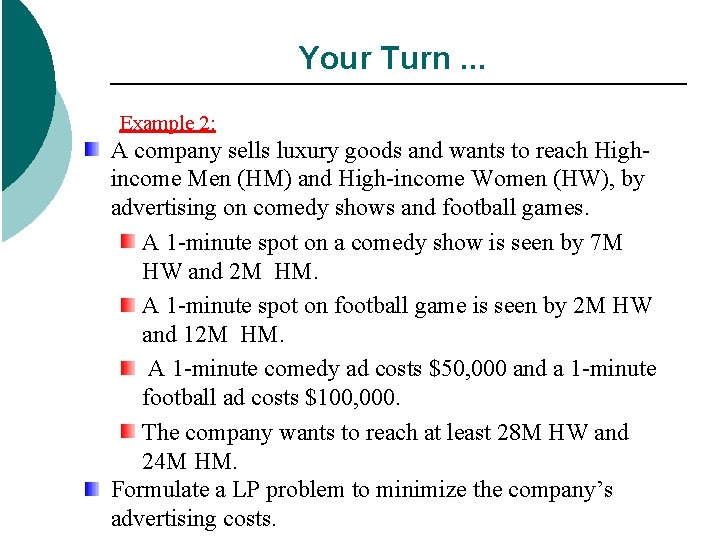Your Turn. . . Example 2: A company sells luxury goods and wants to reach Highincome Men (HM) and High-income Women (HW), by advertising on comedy shows and football games. A 1 -minute spot on a comedy show is seen by 7 M HW and 2 M HM. A 1 -minute spot on football game is seen by 2 M HW and 12 M HM. A 1 -minute comedy ad costs \$50, 000 and a 1 -minute football ad costs \$100, 000. The company wants to reach at least 28 M HW and 24 M HM. Formulate a LP problem to minimize the company’s advertising costs.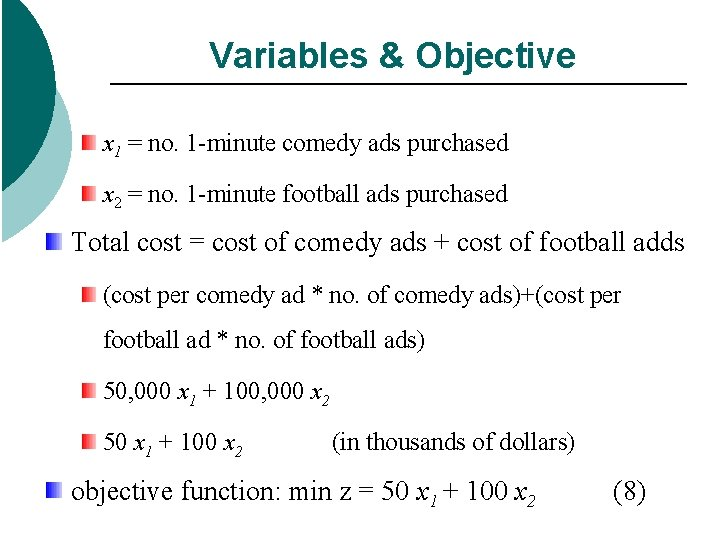Variables & Objective x 1 = no. 1 -minute comedy ads purchased x 2 = no. 1 -minute football ads purchased Total cost = cost of comedy ads + cost of football adds (cost per comedy ad * no. of comedy ads)+(cost per football ad * no. of football ads) 50, 000 x 1 + 100, 000 x 2 50 x 1 + 100 x 2 (in thousands of dollars) objective function: min z = 50 x 1 + 100 x 2 (8)Constraints c 1) must reach at least 28 million HW no. of HW = (HW per comedy add * no. of comedy ads)+ (HW per football add * no. of football ads) = 7 x 1 + 2 x 2 > 28 (9) in millions of viewers c 2) must reach at least 24 million HM no. of HM = (HM per comedy add * no. of comedy ads)+ (HM per football add * no. of football ads) = 2 x 1 + 12 x 2 > 24 (10) sign restrictions: x 1 > 0 ; x 2 > 0; in millions of viewersLP Formulation Min z = 50 x 1 + 100 x 2 s. t. 7 x 1 + 2 x 2 > 28 2 x 1 + 12 x 2 > 24 x 1, x 2 > 0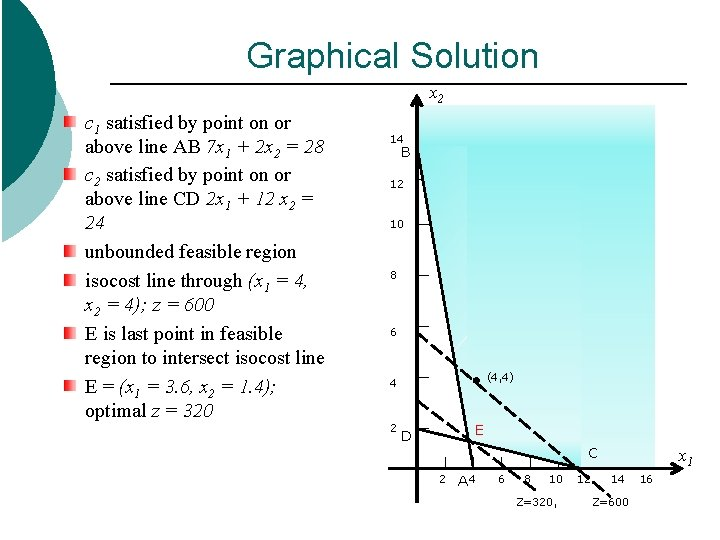Graphical Solution x 2 c 1 satisfied by point on or above line AB 7 x 1 + 2 x 2 = 28 c 2 satisfied by point on or above line CD 2 x 1 + 12 x 2 = 24 unbounded feasible region isocost line through (x 1 = 4, x 2 = 4); z = 600 E is last point in feasible region to intersect isocost line E = (x 1 = 3. 6, x 2 = 1. 4); optimal z = 320 14 B 12 10 8 6 (4, 4) 4 2 E D C 2 A 4 6 8 10 Z=320, 12 x 1 14 Z=600 16A word of caution. . . Some assumptions may not be accurate in the real world. proportionality: e. g. each comedy ad must add exactly 7 M HW and 2 M HM. empirical evidence shows advertising yields diminishing returns after a certain point. additivity: e. g. total viewers = viewers from comedy ads + viewers from football adds. in reality there will be some overlap, same person may see more than 1 type of commercial; so, effectiveness of a comedy ad actually depends on the no. of football ads etc.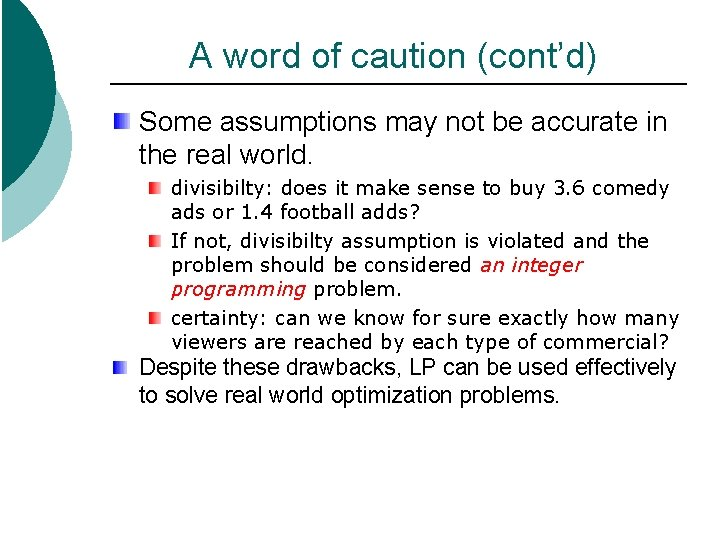A word of caution (cont’d) Some assumptions may not be accurate in the real world. divisibilty: does it make sense to buy 3. 6 comedy ads or 1. 4 football adds? If not, divisibilty assumption is violated and the problem should be considered an integer programming problem. certainty: can we know for sure exactly how many viewers are reached by each type of commercial? Despite these drawbacks, LP can be used effectively to solve real world optimization problems.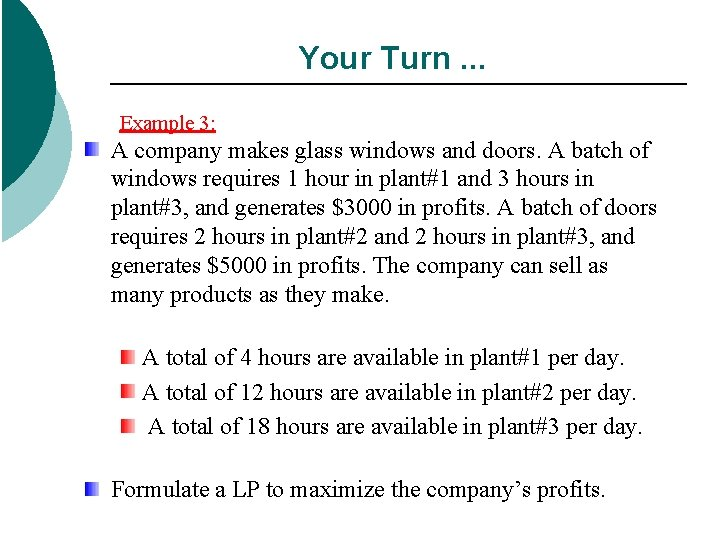Your Turn. . . Example 3: A company makes glass windows and doors. A batch of windows requires 1 hour in plant#1 and 3 hours in plant#3, and generates \$3000 in profits. A batch of doors requires 2 hours in plant#2 and 2 hours in plant#3, and generates \$5000 in profits. The company can sell as many products as they make. A total of 4 hours are available in plant#1 per day. A total of 12 hours are available in plant#2 per day. A total of 18 hours are available in plant#3 per day. Formulate a LP to maximize the company’s profits.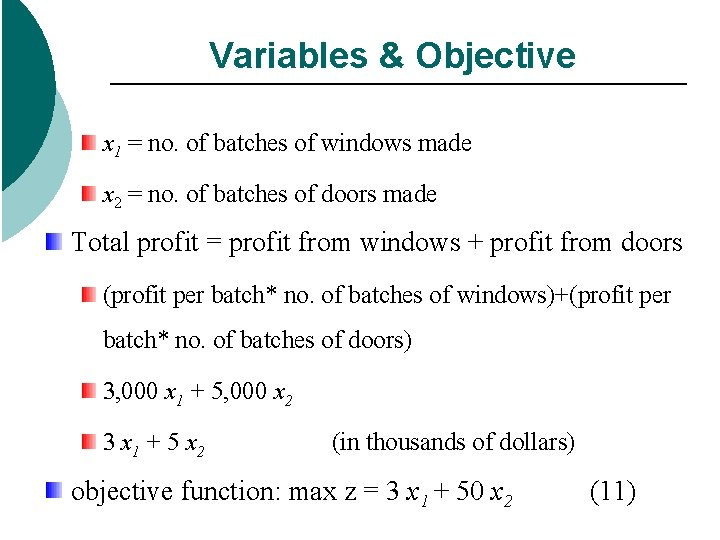Variables & Objective x 1 = no. of batches of windows made x 2 = no. of batches of doors made Total profit = profit from windows + profit from doors (profit per batch* no. of batches of windows)+(profit per batch* no. of batches of doors) 3, 000 x 1 + 5, 000 x 2 3 x 1 + 5 x 2 (in thousands of dollars) objective function: max z = 3 x 1 + 50 x 2 (11)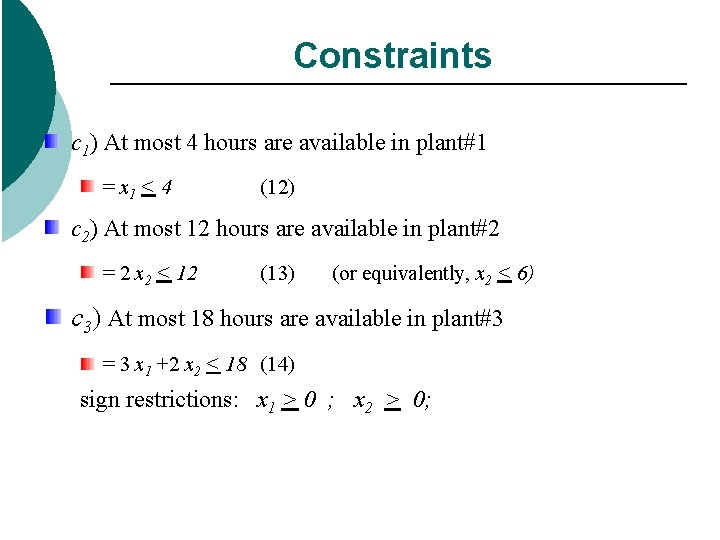Constraints c 1) At most 4 hours are available in plant#1 = x 1 < 4 (12) c 2) At most 12 hours are available in plant#2 = 2 x 2 < 12 (13) (or equivalently, x 2 < 6) c 3) At most 18 hours are available in plant#3 = 3 x 1 +2 x 2 < 18 (14) sign restrictions: x 1 > 0 ; x 2 > 0;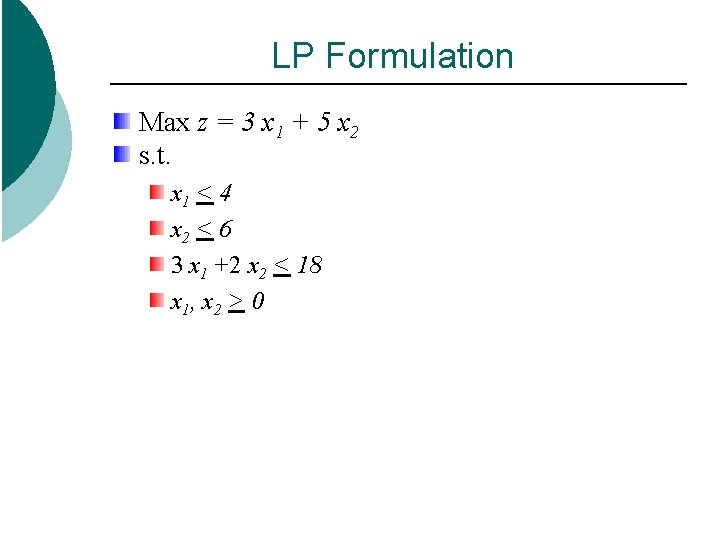LP Formulation Max z = 3 x 1 + 5 x 2 s. t. x 1 < 4 x 2 < 6 3 x 1 +2 x 2 < 18 x 1, x 2 > 0Graphical Solution x 2 c 1 satisfied by point on left of line AB x 1 = 4 c 2 satisfied by point on or below line CD x 2 = 6 c 3 satisfied by point on or below line CD 3 x 1+2 x 2 = 18 bounded feasible region isoprofit line through (x 1 = 0, x 2 = 2); z = 3 x 1+5 x 2 = 10 isoprofit line through (x 1 = 0, x 2 = 4); z = 3 x 1+5 x 2 = 20 G is last point in feasible region to intersect isoprofit line G = (x 1 = 2, x 2 = 6); optimal z = 36 14 B 12 10 F 8 G 6 C D 4 Z=36 2 x 1 2 4 A 6 E 8 10 12 14 16